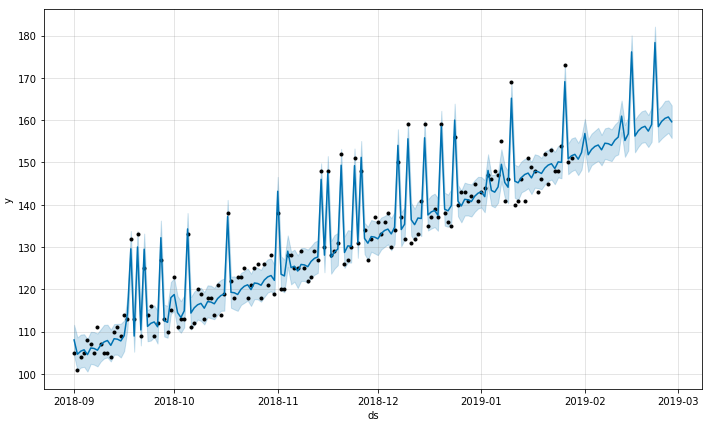1600095300

# Facebook Prophet For Time Series Forecasting in Python

Prophet is an open source time series forecasting algorithm designed by Facebook for ease of use without any expert knowledge in statistics or time series forecasting. Prophet builds a model by finding a best smooth line which can be represented as a sum of the following components:

y(t) = g(t) + s(t) + h(t) + ϵₜ

• Overall growth trend. g(t)
• Yearly seasonality. s(t)
• Weekly seasonality. s(t)
• Holidays effects h(t)

In this series of blog posts, we will see some of the useful functions present in the library `**fbprophet**`listed below with an example.

1. `**Prophet.fit**`
2. `**Prophet.predict**`
3. `**Prophet.plot**`
4. `**Prophet.plot_components**`
5. `**Prophet.add_seasonality**`
6. `**Prophet.add_regressors**`
7. `**Prophet.seasonalities**`
8. `**Prophet.predictive_samples**`

Let’s start by describing the sample data set that we will be using for our demonstration.

#forecasting #python #time-series-forecasting #fbprophet #data-science

## Buddha Community1619518440

## top 30 Python Tips and Tricks for Beginners

Welcome to my Blog , In this article, you are going to learn the top 10 python tips and tricks.

### 8) Check The Memory Usage Of An Object.

#python #python hacks tricks #python learning tips #python programming tricks #python tips #python tips and tricks #python tips and tricks advanced #python tips and tricks for beginners #python tips tricks and techniques #python tutorial #tips and tricks in python #tips to learn python #top 30 python tips and tricks for beginners1619510796

## Lambda, Map, Filter functions in python

Welcome to my Blog, In this article, we will learn python lambda function, Map function, and filter function.

Lambda function in python: Lambda is a one line anonymous function and lambda takes any number of arguments but can only have one expression and python lambda syntax is

Syntax: x = lambda arguments : expression

Now i will show you some python lambda function examples:

#python #anonymous function python #filter function in python #lambda #lambda python 3 #map python #python filter #python filter lambda #python lambda #python lambda examples #python map1600095300

## Facebook Prophet For Time Series Forecasting in Python

Prophet is an open source time series forecasting algorithm designed by Facebook for ease of use without any expert knowledge in statistics or time series forecasting. Prophet builds a model by finding a best smooth line which can be represented as a sum of the following components:

y(t) = g(t) + s(t) + h(t) + ϵₜ

• Overall growth trend. g(t)
• Yearly seasonality. s(t)
• Weekly seasonality. s(t)
• Holidays effects h(t)

In this series of blog posts, we will see some of the useful functions present in the library `**fbprophet**`listed below with an example.

1. `**Prophet.fit**`
2. `**Prophet.predict**`
3. `**Prophet.plot**`
4. `**Prophet.plot_components**`
5. `**Prophet.add_seasonality**`
6. `**Prophet.add_regressors**`
7. `**Prophet.seasonalities**`
8. `**Prophet.predictive_samples**`

Let’s start by describing the sample data set that we will be using for our demonstration.

#forecasting #python #time-series-forecasting #fbprophet #data-science1623226129

## Time Series Analysis Using ARIMA Model With Python

Time series_ is a sequence of time-based data points collected at specific intervals of a given phenomenon that undergoes changes over time. In other words, time series is a sequence taken at consecutive equally spaced points in the time period._

As a example, we can present few time series data sets in different domains such as pollution levels, Birth rates, heart rate monitoring, global temperatures and Consumer Price Index etc. At the processing level, above datasets are tracked, monitored, down sampled, and aggregated over time.

There are different kind of time series analysis techniques in the big data analytical field. Among them few are,

• Autoregression (AR)
• Moving Average (MA)
• Autoregressive Moving Average (ARMA)
• Autoregressive Integrated Moving Average (ARIMA)
• Seasonal Autoregressive Integrated Moving-Average (SARIMA)

ARIMA Model

ARIMA Model is simple and flexible enough to capture relationship we would see in the data and It aims to explain the autocorrelation between the data points using past data. We can decompose the ARIMA model as follow to grab the key elements of it.

• **AR: _Auto regression. _**This is a model that uses the dependent relationship between the data and the lagged data.
• **I:_ Integrated. _**The use of differencing of raw observations (e.g. subtracting an observation from an observation at the previous time step) in order to make the time series stationary.
• **MA: _Moving average. _**A model that uses the relationship between the observations and the residual error from the moving average model applied to lagged observations.

Dataset Explanation

Exploratory Analysis

#python #time-series-analysis #pandas #forecasting #arima #time series analysis using arima model with python1600660800

## Hands-On Guide To Darts - A Python Tool For Time Series Forecasting

Data collected over a certain period of time is called Time-series data. These data points are usually collected at adjacent intervals and have some correlation with the target. There are certain datasets that contain columns with date, month or days that are important for making predictions like sales datasetsstock price prediction etc. But the problem here is how to use the time-series data and convert them into a format the machine can understand? Python made this process a lot simpler by introducing a package called Darts.